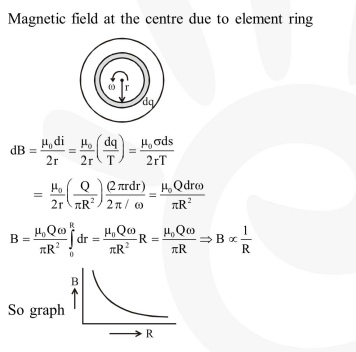Deepak Scored 45->99%ile with Bounce Back Crack Course. You can do it too!

# A charge Q is uniformly distributed over the surface of non-conducting

Question:

A charge $Q$ is uniformly distributed over the surface of non-conducting disc of radius $R$. The disc rotates about an axis perpendicular to its plane

and passing through its centre with an angular velocity $\omega$. As a result of this rotation a magnetic field of induction $B$ is obtained at the centre

of the disc. If we keep both the amount of charge placed on the disc and its angular velocity to be constant and vary the radius of the disc then the

variation of the magnetic induction at the centre of the disc will be represented by the figure :-

1.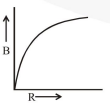2.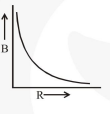3.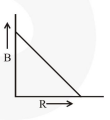4.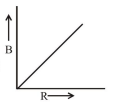Correct Option: , 2

Solution: